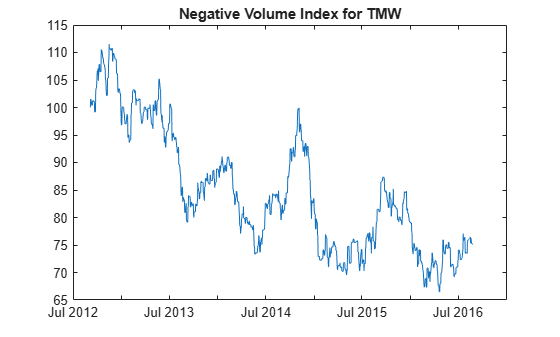# negvolidx

Negative volume index

`negvolidx` has been partially removed and will no longer accept a `fints` object (`tsobj`) argument. Use a matrix, `timetable`, or `table` instead for financial time series.

Use `fts2timetable` to convert a `fints` object to a `timetable` object.

## Syntax

``volume = negvolidx(Data)``
``volume = negvolidx(___,Name,Value)``

## Description

example

````volume = negvolidx(Data)` calculates the negative volume index from the series of closing stock prices and trade volume.```

example

````volume = negvolidx(___,Name,Value)` adds optional name-value pair arguments. ```

## Examples

collapse all

Load the file `SimulatedStock.mat`, which provides a timetable (`TMW`) for financial data for TMW stock.

```load SimulatedStock.mat volume = negvolidx(TMW); plot(volume.Time,volume.NegativeVolume) title('Negative Volume Index for TMW')```## Input Arguments

collapse all

Data with closing prices and trade volume, specified as a matrix, table, or timetable. For matrix input, `Data` is an `M`-by-`2` with closing prices and trade volume stored in the first and second columns. Timetables and tables with `M` rows must contain variables named `'Close'` and `'Volume'` (case insensitive).

Data Types: `double` | `table` | `timetable`

### Name-Value Pair Arguments

Specify optional comma-separated pairs of `Name,Value` arguments. `Name` is the argument name and `Value` is the corresponding value. `Name` must appear inside quotes. You can specify several name and value pair arguments in any order as `Name1,Value1,...,NameN,ValueN`.

Example: ```volume = negvolidx(TMW,'InitialValue',500)```

Initial value for negative volume index, specified as the comma-separated pair consisting of `'InitialValue'` and a scalar positive integer.

Data Types: `double`

## Output Arguments

collapse all

Negative volume index, returned with the same number of rows (`M`) and the same type (matrix, table, or timetable) as the input `Data`.

collapse all

### Negative Volume Index

Negative volume index shows the days when the trading volume of a particular security is substantially lower than other days.

 Achelis, S. B. Technical Analysis from A to Z. Second Edition. McGraw-Hill, 1995, pp. 193–194.